#### Returning to the system vector fields

Now the formal algebra concepts can be applied to the steering problem. The variables become the system vector fields: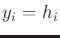for allfromto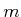. For the P. Hall basis elements, each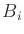becomes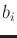. The Lie group becomes the state space, and the Lie algebra is the familiar Lie algebra over the vector fields, which was introduced in Section 15.4.3. Consider how an element of the Lie group must evolve over time. This can be expressed using the differential equation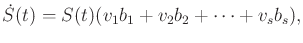(15.128)

which is initialized with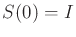. Here,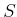can be interpreted as a matrix, which may, for example, belong to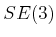.

The solution at every time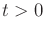can be written using the Chen-Fliess series, (15.127):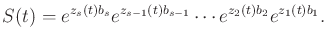(15.129)

This indicates that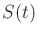can be obtained by integrating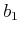for time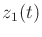, followed by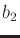for time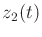, and so on until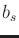is integrated for time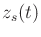. Note that the backward P. Hall coordinates now vary over time. If we determine how they evolve over time, then the differential equation in (15.128) is solved.

The next step is to figure out how the backward P. Hall coordinates evolve. Differentiating (15.129) with respect to time yields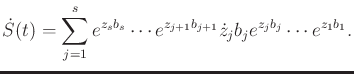(15.130)

Steven M LaValle 2020-08-14